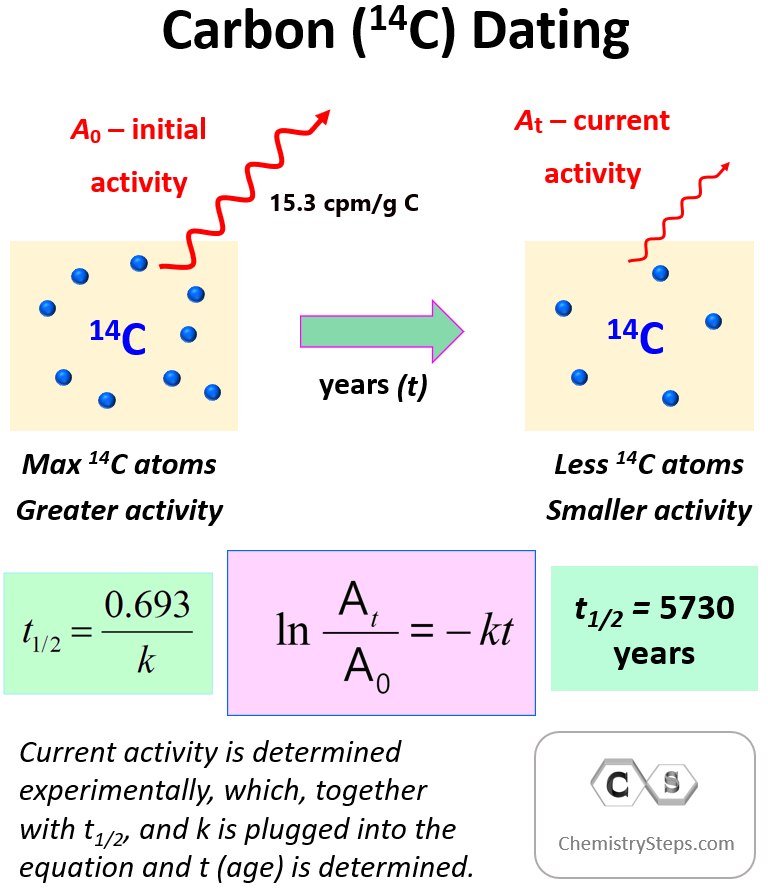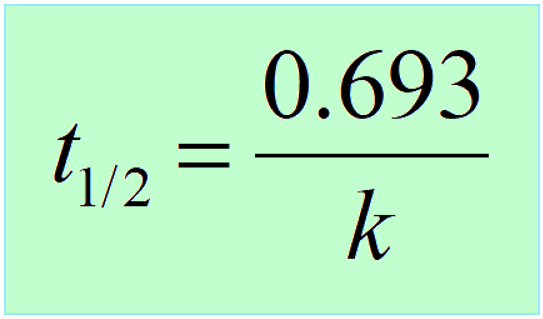\$referrer_host = www.google.com. Carbon Dating Practice Problems - Chemistry Steps

## General Chemistry

Carbon dating is a technique that measures the age of ancient objects containing carbon.

The 14C isotope of carbon is radioactive and is only accumulated in living organisms.Once dead, the amount of 14C, and thus its ratio to the 12C decreases which results in decreasing the radioactivity of the sample.To determine the age of the sample, we need to compare the initial (15.3 cpm/g C) and current activity of 14C using the integrated rate law of first-order reactionswhere At is the current activity, A0 is the initial activity, k is the rate (decay) constant, and t is the time for which the decay is measured.

It is important to remember that instead of activity (A), it can be the mass (m), moles, number of atoms (N), etc.

Another key component is the half-life, which, remember is constant for first-order reactions, and is correlated to the rate constant of the process:Carbon dating is a specific example of using the kinetics of nuclear reactions. For a more comprehensive list of the half-life, and other features of nuclear reactions, check the following set of practice problems:

#### Practice

1.

An archaeological sample gives 4.80 disintegrations of 14C per minute per gram of total carbon. How old is the sample if the initial decay rate of  14C is 15.3 cpm/g C, and its half-life is 5730 years?

9.59 x 103 years

Solution

We are going to use the integrated rate law for first-order reactions to determine the age of the sample (t).

$\ln \;\frac{{{A_t}}}{{ {A_0}}}\; = \; – kt$

${\rm{ln}}\;\frac{{{\rm{4}}{\rm{.80}}}}{{{\rm{ 15}}{\rm{.3}}}}\;{\rm{ = }}\;{\rm{ – }}\frac{{{\rm{0}}{\rm{.693}}}}{{{\rm{5730}}\;{\rm{yrs}}}}\, \times \,t$

-1.159237 = -0.000120942 t

t = 9.59 x 103 years

2.

How old is an ancient painting with a 14C activity of 7.60 cpm/g if the initial decay rate of  14C is 15.3 cpm/g C, and its half-life is 5730 years?

5.79 x 103 years

Solution

We are going to use the integrated rate law for first-order reactions to determine the age of the sample (t).

$\ln \;\frac{{{A_t}}}{{ {A_0}}}\; = \; – kt$

${\rm{ln}}\;\frac{{{\rm{7}}{\rm{.60}}}}{{{\rm{ 15}}{\rm{.3}}}}\;{\rm{ = }}\;{\rm{ – }}\frac{{{\rm{0}}{\rm{.693}}}}{{{\rm{5730}}\;{\rm{yrs}}}}\, \times \,t$

-0.699704 = -0.000120942 t

t = 5.79 x 103 years

3.

A wooden tray discovered by a group of archeologists has a 14C activity that is 73% of the current 14C activity. How old is the tray?

2.60 x 103 years

Solution

73% of the current activity means A = 0.73 A0, and this is what we will use in the formula:

$\ln \;\frac{{{A_t}}}{{ {A_0}}}\; = \; – kt$

$\ln \;\frac{{0.73\,{A_0}}}{{ {A_0}}}\; = \; – kt$

${\rm{ln}}\;{\rm{0}}{\rm{.73}}\;{\rm{ = }}\;{\rm{ – }}\frac{{{\rm{0}}{\rm{.693}}}}{{{\rm{5730}}\;{\rm{yrs}}}}\, \times \,t$

-0.3147107 = -0.000120942 t

t = 2.60 x 103 years

4.

The activity of 400. mg sample of 14C carbon collected from an ancient cloth is 145 disintegrations per hour. How old is the cloth if the activity of a current 1.00-g sample of carbon is 921 disintegrations per hour?

7.71 x 103 years

Solution

We need to follow the same steps in this problem as we have been doing so far. The only difference is that the masses of samples are not equal, and therefore, we need to calibrate them. Let’s adjust the activity of the collected sample to 1.00 g. 400. mg is 0.400 g, so, to find the activity of the old sample per gram of caron, we need to divide 145 by 0.400:

A = 145 ÷ 0.400 = 362.5 dis/min

And now we can use the integrated rate law to determine the age of the sample:

$\ln \;\frac{{{A_t}}}{{ {A_0}}}\; = \; – kt$

${\mathop{\rm l}\nolimits} {\rm{n}}\;\frac{{{\rm{362}}{\rm{.5}}}}{{{\rm{ 921}}}}\;{\rm{ = }}\;{\rm{ – }}\frac{{{\rm{0}}{\rm{.693}}}}{{{\rm{5730}}\,{\rm{yrs}}}}t$

-0.932436 = -0.000120942 t

t = 7.71 x 103 years

5.

The bones of a camel were found to have 14C activity of 3.40 dis/min · g carbon. Determine, approximately, how long ago the animal lived given the initial decay rate of  14C is 15.3 cpm/g carbon, and its half-life is 5730 years?

1.24 x 104 years

Solution

We are going to use the integrated rate law for first-order reactions to determine the age of the animal (t).

$\ln \;\frac{{{A_t}}}{{ {A_0}}}\; = \; – kt$

${\rm{ln}}\;\frac{{{\rm{3}}{\rm{.40}}}}{{{\rm{ 15}}{\rm{.3}}}}\;{\rm{ = }}\;{\rm{ – }}\frac{{{\rm{0}}{\rm{.693}}}}{{{\rm{5730}}\;{\rm{yrs}}}}\, \times \,t$

-1.504077 = -0.000120942 t

t = 1.24 x 104 years

1686015468